Type 2 pseudo amino acid composition
Type 2 PseAA composition is also called the series-correlation type and generates 20 + i*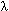discrete numbers to represent a protein (i is the number of amino acid attributes selected), which was introduced by Prof. Kuo-Chen Chou in 2005 and the related publications are:

(1) Chou, K.C. (2005). Using amphiphilic pseudo amino acid composition to predict enzyme subfamily classes, Bioinformatics, 21, 10-19.
(2) Chou,K.C. and Cai Y.D. (2005). Prediction of membrane protein types by incorporating amphipathic effects, J Chem Inf Model, 45(2):407-13

The essence of pseudo-amino acid composition is, on one hand, to include the main feature of amino acid composition, but on the other, to include information beyond amino acid composition. The conventional amino acid composition contains 20 components, or discrete numbers, each reflecting the occurrence frequency of one of the 20 native amino acids in a protein. For the pseudo-amino acid composition, however, there are some other elements in addition to the 20 components. It is through these additional discrete numbers that the sequence order effect of a protein is approximately reflected and improvements are made, as will be shown below. The basic ideas of Type 2 pseudo amino acid composition is as following:
Consider a protein chain of L amino acid residues: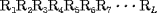(1)
where R1 represents the residue at sequence position 1, R2 represents the residue at position 2, and so forth. Since the amphipathic feature of a protein is mainly reflected by the hydrophobicity and hydrophilicity of its constituent amino acids, their indexes will be used to formulate the sequence-order correlated factors (Figure 2) through the following equations: where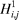and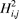are the hydrophobicity and hydrophilicity correlation functions given by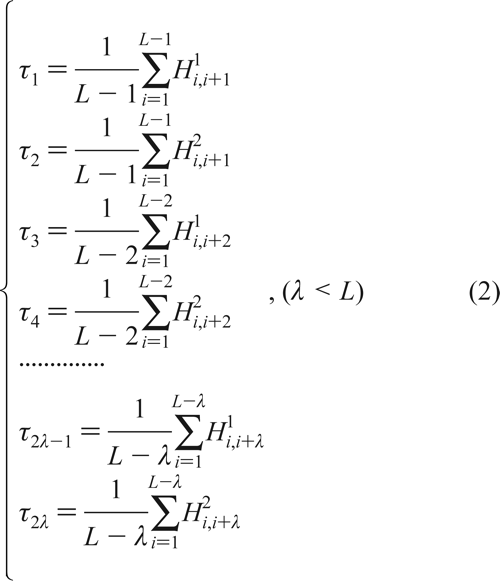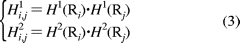where H1(Ri) and H2(Ri) are respectively the hydrophobicity and hydrophilicity values for the ith (i = 1, 2, ..., L) amino acid in eq 1, and the dot (.) means the multiplication sign. Note that before substituting the values of hydrophobicity and hydrophilicity into eq 3, they were all subjected to a standard conversion as described by the following equation: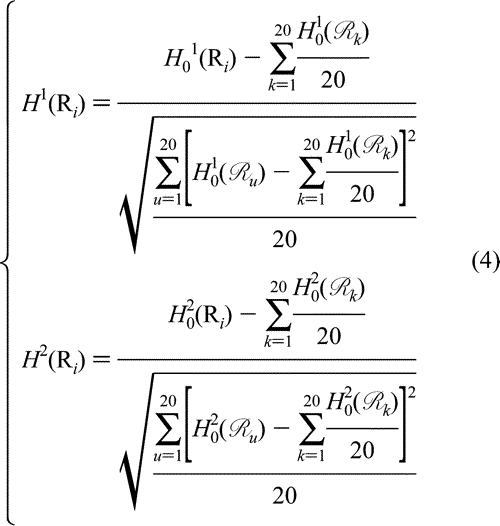where we use Ri (i = 1, 2, ..., 20) to represent the 20 native amino acids according to the alphabetical order of their single-letter codes: A, C, D, E, F, G, H, I, K, L, M, N, P, Q, R, S, T, V, W, and Y. The symbols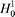and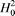represent the original hydrophobicity and hydrophilicity values for the amino acid in the follow-up brackets. The advantage to use the converted hydrophobicity and hydrophilicity values obtained via eq 4 is that they will have a zero mean value over the 20 native amino acids and will remain unchanged if going through the same conversion procedure again. After incorporating the sequence-order correlated factors from eq 2 into the classical 20D (dimensional) amino acid composition, we obtain a pseudo amino acid composition with (20 + 2) components. In other words, the representation for a protein sample P is now formulated as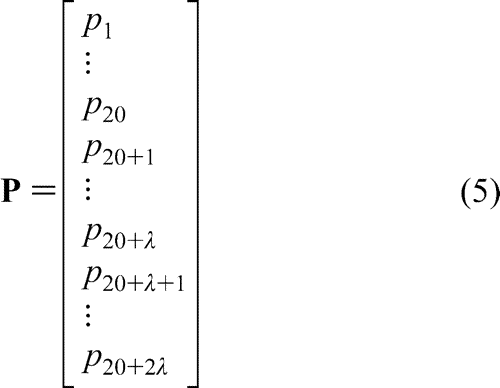where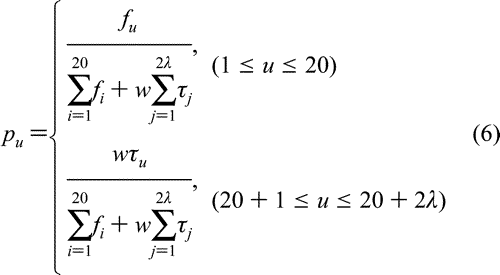where fi (i = 1, 2, ..., 20) are the normalized occurrence frequencies of the 20 amino acids in the protein P, j is the j-tier sequence-correlation factor computed according to eq 2, and w is the weight factor. As we can see from eqs 5-6, the first 20 components reflect the effect of the classical amino acid composition, while the components from 20 + 1 to 20 + 2reflect the amphipathic sequence-order pattern. A set of such 20 + 2components is called the "amphipathic pseudo amino acid composition".